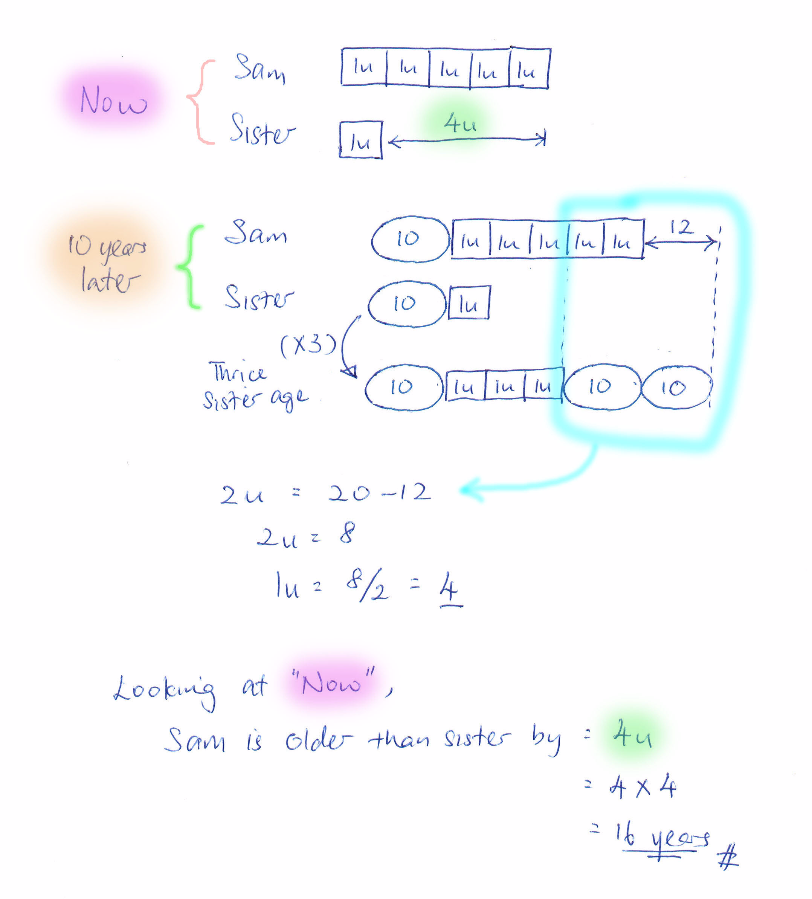# Question

Sam is 5 times as old as his sister. In 10 years time, Sam will be 12 years less than thrice his sister’s age then. How much older is Sam than his sister?

Source: Nan Chiau Primary

Now

Sam                          X  X  X  X  X  [5u]

Sister                        X                     [u]

10 yrs later

Sam                10 +   X  X  X  X  X—-> (1) [10+5u] This sum is 12 yrs less than (2)

Sister              10 +   X

(3x Sister)     30 +  X  X X ——–> (2) [30+3u]

By cancelling out “X X X” in the model:

Comparing (1) and (2): 10 + X +X + 12 = 30 –> X=4

By equations:

Comparing (1) and (2): [10 + 5u] + 12 = [30+3u] –> u=4

Sam is 16 years older than his sister now (difference of 4u = 16)#

Checking:

Now                 +10 yrs later

Sam            20                  30

Sister           4                   14

3x Sister                           42 (12 more than Sam)

0 Replies 2 Likes ✔Accepted Answer

16 years older

0 Replies 0 Likes0 Replies 2 Likes